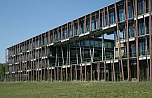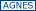# Humboldt-Universität zu BerlinMath.- Nat. FakultätInstitut für Physikin english

Sommersemester 2022
Stand: 04.08.22 14:05:32

_### 4020220122 Stochastische ThermodynamikVL Mo 11-13 wöch. NEW 15 2'101 (24) Mehrnaz Anvari, Jürgen Kurths

Digital- & Präsenz-basierter Kurs

Unterrichtssprache
DE
Voraussetzungen
Thermodynamics
Gliederung / Themen / Inhalte
The course is divided to two parts. In the first part the concept of stochastic process, including continuous and discontinuous Markov processes, is
introduced. In the second part stochastic thermodynamics will be introduced, including the fundamental thermodynamics laws and the non-equilibrium thermodynamics concept in stochastic processes.

Course content
I. Stochastic Process (6 sessions)
§ Lecture 1: Introduction
- Brownian motion
§ Lecture 2: Continuous Markov Process
- Fokker-Planck equation
- Langevin equation
- Chapman-Kolmogorov equation
- Application: Turbulence, Earthquake
§ Lecture 3: Discontinuous Markov process I
- Differential Chapman-Kolmogorov (Master equation)
- Kramers-Moyal equation
- Pawula theorem
§ Lecture 4: Discontinuous Markov process II
- Jump-Diffusion equation
- Example: Epilepsy
II. Stochastic Thermodynamics (9 sessions)
§ Lecture 1: Equilibrium thermodynamics
- Review of equilibrium thermodynamics
§ Lecture 2: violation of second law of thermodynamics
- The perpetuum mobile of the second kind
- Maxwell’s Demon
- Feynman’s ratchet
- Szilard’s engine
§ Lecture 3: Thermodynamics laws for stochastic processes
- First law of thermodynamics for Fokker-Planck equation
- Second law of thermodynamics for Fokker-Planck equation
- Example: Colloidal particle
§ Lecture 4: Nonequilibrium thermodynamics I
- Detailed balance
- Fluctuation theorem, Detailed Fluctuation theorem, Integral
Fluctuation theorem